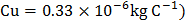# The equivalent resistance between points A and B of an infinite network of resistances, each of 1, connected as shown isa) Infinite b) 2c)d) zero

## Question ID - 151505 :- The equivalent resistance between points A and B of an infinite network of resistances, each of 1, connected as shown isa) Infinite b) 2c)d) zero

3537

(c)

Letbe the equivalent resistance of entire network between A and B. Hence, we haveresistance of parallel combination of 1andNext Question :
 If 100 kWh of energy is consumed at 66 V in a copper voltmeter, then the mass of copper liberated will be (Given ,ECE ofa) 1.65 kg b) 1.8 kg c) 3.3 kg d) 3.6 kg